• ## MATLAB立体绘图

千次阅读 2020-03-09 10:16:00
MATLAB绘图基础2 MATLAB立体绘图2.1 生成网格坐标矩阵的函数2.2 画三维曲面的函数2.3 三维旋转体的绘制柱面球面2.4 三维图形的处理视点处理色彩处理消隐处理裁剪处理❤隐函数作图 2 MATLAB立体绘图 2.1 生成...


MATLAB绘图基础
2 MATLAB的立体绘图2.1 生成网格坐标矩阵的函数2.2 画三维曲面的函数2.3 三维旋转体的绘制柱面图球面图
2.4 三维图形的处理视点处理色彩处理消隐处理裁剪处理

❤隐函数作图

2 MATLAB的立体绘图
2.1 生成网格坐标矩阵的函数
[X,Y]=meshgrid(x,y)生成X-Y平面的网格坐标矩阵[X,Y,Z]=sphere(n)生成球面的三维坐标矩阵[X,Y,Z]=cylinder(r,n)生成柱面的三维坐标矩阵[X,Y]=meshgrid(x,y);Z=peaks(X,Y)peaks多峰函数，生成多峰曲面的坐标矩阵
例子：
%例一
theta=0:pi/50:6*pi;
x=cos(theta); y=sin(theta); z=0:300;
plot3(x,y,z);
%例二
x=-3:0.1:3; y=-3:0.1:3;
[X,Y]=meshgrid(x,y); Z=X.^2+Y.^2;
surf(X,Y,Z);

2.2 画三维曲面的函数
plot3(X,Y,Z)三维曲面mesh(X,Y,Z)三维网格曲面meshz(X,Y,Z)可将曲面加上围裙meshc(X,Y,Z)同时画出网状图与等高线surf(X,Y,Z)三维填充曲面（更精细）surfc(X,Y,Z)同时画出曲面图与等高线bar3(A)三维柱状图stem3(A)三维棒状图pie3(A)三维饼状图fill3(A)三维填充图waterfall(X,Y,Z)三维瀑布图，可在x方向或y方向产生水流效果contour3(X,Y,Z)三维等高线图contour(X,Y,Z)画出曲面等高线在XY平面的投影
例子：
%mesh和plot是三度空间立体绘图的基本命令，mesh可画出立体网状图，plot则可画出立体曲面图，两者产生的图形都会依高度而有不同颜色。
%plot3可画出三度空间中的曲线。
t=linspace(0,20*pi, 501);
subplot(3,4,1),plot3(t.*sin(t), t.*cos(t), t);title('plot3');
%也可同时画出两条三度空间中的曲线
t=linspace(0, 10*pi, 501);
subplot(3,4,2),plot3(t.*sin(t), t.*cos(t), t, t.*sin(t), t.*cos(t), -t);title('plot3');
%mesh可画出立体网状图。
x=linspace(-2, 2, 25); %在x轴上取25点
y=linspace(-2, 2, 25); %在y轴上取25点
[xx,yy]=meshgrid(x, y); %xx和yy都是21x21的矩阵
zz=xx.*exp(-xx.^2-yy.^2); %计算函数值，zz也是21x21的矩阵
subplot(3,4,3),mesh(xx, yy, zz);title('mesh'); %画出立体网状图
%peaks函数可产生一个凹凸有致的曲面，包含了三个局部极大点及三个局部极小点。
subplot(3,4,4),peaks;
%meshz可将曲面加上围裙。
[x,y,z]=peaks;
subplot(3,4,5),meshz(x,y,z);title('meshz');
axis([-inf inf -inf inf -inf inf]);
%meshc同时画出网状图与等高线。
[x,y,z]=peaks;
subplot(3,4,6),meshc(x,y,z);title('meshc');
axis([-inf inf -inf inf -inf inf]);
%surf画出立体曲面图
x=linspace(-2, 2, 25); %在x轴上取25点
y=linspace(-2, 2, 25); %在y轴上取25点
[xx,yy]=meshgrid(x, y); %xx和yy都是21x21的矩阵
zz=xx.*exp(-xx.^2-yy.^2); %计算函数值，zz也是21x21的矩阵
subplot(3,4,7),surf(xx, yy, zz);title('surf'); %画出立体曲面图
%surfc同时画出曲面图与等高线。
[x,y,z]=peaks;
subplot(3,4,8),surfc(x,y,z);title('surfc');
axis([-inf inf -inf inf -inf inf]);
%waterfall可在x方向或y方向产生水流效果。
[x,y,z]=peaks;
subplot(3,4,9),waterfall(x,y,z);title('waterfall-x');
axis([-inf inf -inf inf -inf inf]);
%在y方向产生水流效果
[x,y,z]=peaks;
subplot(3,4,10),waterfall(x',y',z');title('waterfall-y');
axis([-inf inf -inf inf -inf inf]);
%contour3画出曲面在三度空间中的等高线。
subplot(3,4,11),contour3(peaks, 20);title('contour3');
axis([-inf inf -inf inf -inf inf]);
%contour画出曲面等高线在XY平面的投影。
subplot(3,4,12),contour(peaks, 20);title('contour');2.3 三维旋转体的绘制
柱面图
由cylinder函数实现，调用格式为：
[x,y,z]=cylinder(R,n); %其中R是一个向量，存放柱面各个等间隔高度上的半径，n表示在圆柱圆周上有n个间隔点，默认有20个间隔点。
如：cylinder(3)生成一个圆柱，cylinder([10,1])生成一个圆锥，而t=0:pi/100:4*pi; R=sin(t); cylinder(R,30);生成一个正弦圆柱面。
[X,Y,Z]=cylinder(R)或[X,Y,Z]=cylinder此形式为默认N=20且R=[1 1]

例子：
t=0:pi/20:2*pi;
[x,y,z]=cylinder(2+sin(t),30);
subplot(1,3,1);surf(x,y,z);
axis('equal');axis('square'); %控制坐标轴的大小相同
subplot(1,3,2);
x=0:pi/20:pi*3;
r=5+cos(x);
[a,b,c]=cylinder(r,30);
mesh(a,b,c);
axis('equal');axis('square');
subplot(1,3,3);
r=abs(exp(-0.25*t).*sin(t));
t=0:pi/12:3*pi;
r=abs(exp(-0.25*t).*sin(t));
[X,Y,Z]=cylinder(r,30);
mesh(X,Y,Z);
colormap([1 0 0]);
axis('equal');axis('square');球面图
由sphere函数实现，调用格式为：
[X,Y,Z]=sphere(N); %此函数生成3个(N+1)*(N+1)的矩阵,利用函数surf(X,Y,Z) 可绘制出圆心位于原点、半径为1的单位球体。
n决定了球面的圆滑程度，其默认值为20。若n值取的比较小，则绘制出多面体的表面图。
[X,Y,Z]=sphere; %此形式使用了默认值N=20。
sphere(N); %只是绘制了球面图而不返回任何值。

例子：
subplot(1,2,1);
[x,y,z]=sphere;
surf(x,y,z);
axis('equal');axis('square'); %控制坐标轴的大小相同
subplot(1,2,2);
[a,b,c]=sphere(40);
t=abs(c);
surf(a,b,c,t);
axis('equal');axis('square');
colormap('hot');2.4 三维图形的处理
视点处理
设置视点的函数view，调用格式为：
view(az,el); %其中az为方位角，el为仰角，它们均以度为单位。系统默认的视点定义为方位角为-37.5度，仰角30度。

例子：
subplot(2,2,1);mesh(peaks);view(-37.5,30);title('-37.5°,30°');
subplot(2,2,2);mesh(peaks);view(0,90);title('0°,90°');
subplot(2,2,3);mesh(peaks);view(90,0);title('90°,0°');
subplot(2,2,4);mesh(peaks);view(-7,-10);title('-7°,-10°');色彩处理
内建矩阵
colormap hot

三维图形表面的着色
shading faceted; %将每个网格片用其高度对应的颜色进行着色，网格线是黑色

消隐处理
例子：
z=peaks(50);
subplot(2,1,1);
mesh(z);title('消隐前的网图')
hidden off
subplot(2,1,2)
mesh(z);title('消隐后的网图')
hidden on
colormap([0 0 1]);裁剪处理
将图形中需要裁剪部分对应的函数值设置成NaN，使函数值为NaN的部分将不显示出来，从而达到对图形进行裁剪的目的。 例子：
%削掉正弦波顶部或底部大于0.5的部分。
subplot(1,2,1);
x=0:pi/10:4*pi;
y=sin(x);
i=find(abs(y)>0.5);
x(i)=NaN;
plot(x,y);
axis([0 15 -0.4 0.4]);axis square
%绘制两个球面，其中一个在另一个里面，将外面的球裁掉一部分，以便能看到里面的球。
subplot(1,2,2);
[x,y,z]=sphere(25);%生成外面的大球
z1=z;
z1(:,1:4)=NaN;%将大球裁去一部分
c1=ones(size(z1));
surf(3*x,3*y,3*z1,c1);       %生成里面的小球
hold on
z2=z;
c2=2*ones(size(z2));
c2(:,1:4)=3*ones(size(c2(:,1:4)));
surf(1.5*x,1.5*y,1.5*z2,c2);
colormap([0 1 0;0.5 0 0;1 0 0]);
grid on;hold off;axis equal❤隐函数作图
Matlab提供了ezplot函数绘制隐函数图形，用法如下： ①对于函数f=f(x)，ezplot的调用格式为：
ezplot(f); %在默认区间(-2pi,2pi)绘制图形
ezplot(f,[a,b]); %在区间(a,b)绘制图形

②对于隐函数f=f(x,y)，ezplot的调用格式为：
ezplot(f); %在默认区间(-2pi,2pi)绘制f(x,y)=0的图形
ezplot(f,[xmin,xmax,ymin,ymax]); %在区间[xmin,xmax,ymin,ymax]绘制图形
ezplot(f,[a,b]); %在区间(a,b)绘制图形

③对于参数方程x=x(t)，y=y(t)，ezplot函数的调用格式为：
ezplot(x,y); %在默认区间绘制x=x(t),y=y(t)图形
ezplot(x,y,[tmin,tmax]); %在区间(tmin,tmax)绘制x=x(t),y=y(t)图形

其他隐函数绘图还有ezpolar，ezcontour，ezplot3，ezmesh，ezmeshc，ezsurf，ezsurfc。 例子：
subplot(2,2,1);
ezplot('x^2+y^2-9');axis equal;
subplot(2,2,2);
ezplot('x^3+y^3-5*x*y+1/5')
subplot(2,2,3);
ezplot('cos(tan(pi*x))',[0,1]);
subplot(2,2,4);
ezplot('8*cos(t)','4*sqrt(2)*sin(t)',[0,2*pi]);展开全文• matlab开发-三维立体图。使用surf命令在3D中绘制X-Y-Z数据。不需要曲线拟合工具箱。
• matlab程序实现3d笛卡尔心形图案，可以作为一个小玩意送给你喜欢的妹子，理科生的烂漫，里面是代码
• ## matlab三维立体图

千次阅读 2017-08-02 14:48:51
1. 三维立体图的基本要件： 全空间网格化网格节点的物理属性值 2.数据准备 数据不易贴，我放在了百度网盘：点击下载数据 大概如下形式： TIP: 这里的数据矩阵为v(5276),可以看成一本27页纸，每页...

前言：在地球物理勘探，流体空间分布等多种场景中，定位空间点P（x,y,x）的物理属性值Q，并绘制三维空间分布图，对我们洞察空间场景有十分重要的意义。
1. 三维立体图的基本要件：
全空间网格化网格节点的物理属性值
2.数据准备
数据不易贴，我放在了百度网盘：点击下载数据 大概如下形式：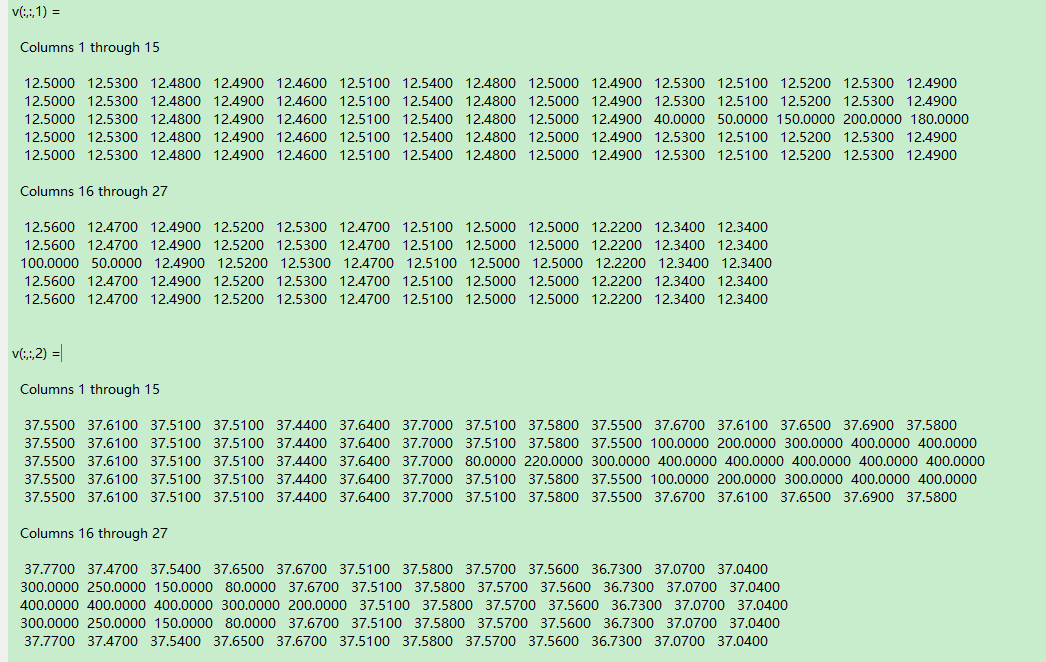TIP: 这里的数据矩阵为v(5276),可以看成一本27页纸，每页绘制了5*6的网格，然后27页纸叠在一起。当你理解本图绘制后，数据可以随意制作。

3.主要函数：slice、isosurface、patch

NOTE:想了解每个函数的用法可以在matlab command windows中 输入 doc slice即可获得slice的matlab详解

4.主要代码：

TIP：你可以将代码复制到一起运行，此处我是单独展示，分别绘图

1.单独切片，切法可自定义。便于展示局部细节

clc,clear
v=reshape(v,5,27,6);
[x,y,z]=meshgrid(1:27,1:5,1:6);
h=figure(1);
set(h,'name','取单切片')
subplot(221)
slice(x,y,z,v,[],,[]);
set(gca,'zdir','reverse');
axis equal
grid on
subplot(222)
slice(x,y,z,v,[],,[]);
colormap('jet')
set(gca,'zdir','reverse');
axis equal
grid on
subplot(223)
slice(x,y,z,v,[],,[]);
set(gca,'zdir','reverse');
axis equal
grid on
subplot(224)
slice(x,y,z,v,[],,[]);
set(gca,'zdir','reverse');
axis equal
grid on

效果图：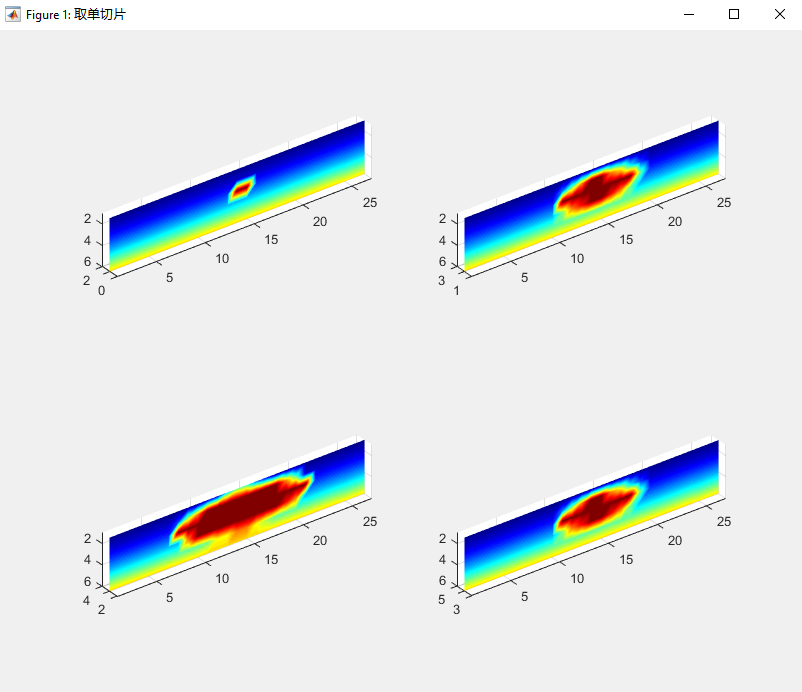2.全空间立体切片

h2=figure(2);
slice(x,y,z,v,[1:2:27],[2 3 4],[2 3 4 5])
colorbar
colormap('jet')
set(gca,'zdir','reverse');
axis equal
grid on
box on

效果图：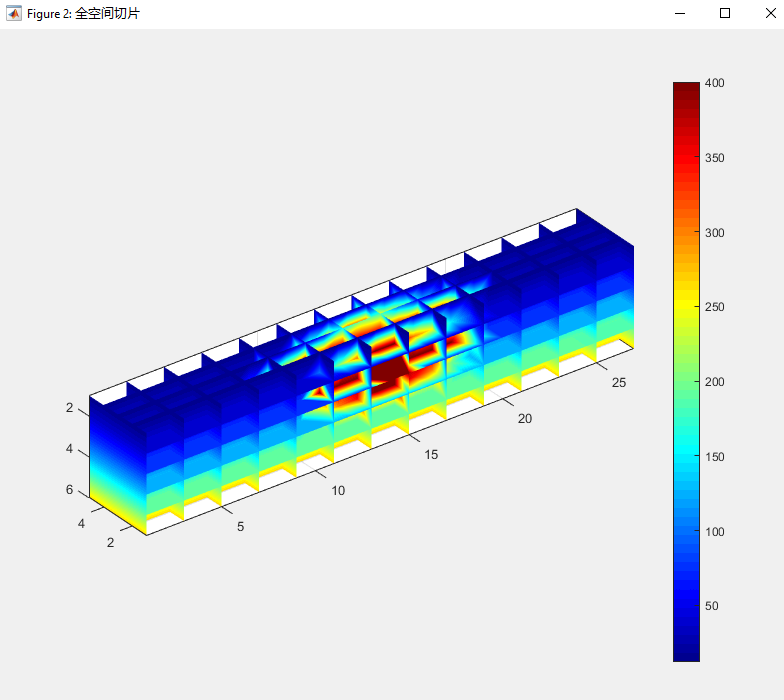3.立体包络图

h3=figure(3);
set(gcf,'InvertHardcopy','off')
fw=350;                                                                                                   %%此值为最外层包络面取值
fv=isosurface(x,y,z,v,fw);
p=patch(fv);
set(p,'facecolor','b','edgecolor','none');
patch(isocaps(x,y,z,v, fw), 'FaceColor', 'interp', 'EdgeColor', 'none');
colorbar
colormap('jet')
box on
daspect([1,1,1])
view(3)
set(gca,'zdir','reverse','color',[0.2,0.2,0.2]);
camlight
camproj perspective
lighting phong
axis equal
grid on
title(['最外层表面的值为： ' , num2str(fw)]);

NOTE：上述代码中的fw是作主要可变参数，控制最外层包络面的值
如下演示多个fw下不同的效果图：csdn博客与此同步，欢迎转载，注明出处。有任何问题和建议，欢迎留言讨论，也可以发我邮箱wenbya@foxmail.com

展开全文• 最近在实验室研究有关立体图像舒适度的项目，简单研究了一下怎么获得视差的程序代码。
• 从当前的 3D 图形创建一个红蓝立体图。 双目旋转角度可以改变。 H=snaprb(alpha) alpha：双目旋转角度。 默认情况下，使用 alpha=1 deg 并增加深度效果的值。 例子： [X,Y,Z] = 峰值（30）； 网格（X，Y，Z，'...
• 文件里面的.mat文件就是参考，要矫正自己的必须要先得到标定结果，就是你自己相机标定的结果.mat文件
• 3 绘制图形句柄 就是绘制小型立方体，中型立方体和大型立方体，其中鼠标移动到中型立方体中心时中型立方体变成大型立方体，这个可以靠设置图形对象的XData,YData,ZData数值来改变 3.1 构造网格 由于surf曲面可以...
效果教程部分
1 图片导入与大小重设
需要有一个名为album的文件夹和当前m文件在同一文件夹，另外ablum文件夹内至少要有一张jpg格式图片
path='.\album\';%文件夹名称
files=dir(fullfile(path,'*.jpg'));
picNum=size(files,1);

%遍历路径下每一幅图像
for i=1:picNum
fileName=strcat(path,files(i).name);
img=imresize(img,[120,120]);
imgSet{i}=img;
end

我们注意到，这里用了一次imresize将突破变为120x120大小，这里重设大小有三个作用：
将不是方形的图片变为方形将图像设置固定大小，方便构造网格放置图片120x120的大小大约是能让图片表示清晰为前提下最小的大小，图片太大的话运行会卡，太小的话不清晰
2 fig axes设置
% fig axes设置
fig=figure('units','pixels','position',[50 50 600 600],...
'Numbertitle','off','resize','off',...
ax=axes('parent',fig,'position',[-0.5 -0.5 2 2],...
'XLim', [-6 6],...
'YLim', [-6 6],...
'ZLim', [-6 6],...
'Visible','on',...
'XTick',[], ...
'YTick',[],...
'Color',[0 0 0],...
'DataAspectRatioMode','manual',...
'CameraPositionMode','manual');
hold(ax,'on')

大部分设置大家都能看懂，这里讲解一下一些比较少见的设置：
2.1 为什么 axes的’position’属性不设置[0 0 1 1]?

因为是3D坐标轴，设置为[0 0 1 1]后旋转起来效果是这样的，所以我们axes要设置的比figure大一圈：2.2 为什么要设置CameraPositionMode这一奇怪的属性？

因为我们后期要频繁改变CameraPosition这一属性，而CameraPositionMode设置为manual可以让视角完全按照CameraPosition的数值来调整，至于为什么要调整视角呢？ , 当然是因为如果对图像位置数据进行处理数据量会贼大，因此我们不妨直接转动axes视角而非转动图片。

3 绘制图形句柄
就是绘制小型立方体，中型立方体和大型立方体，其中鼠标移动到中型立方体中心时中型立方体变成大型立方体，这个可以靠设置图形对象的XData,YData,ZData数值来改变
3.1 构造网格
由于surf曲面图可以将图像贴在上面，还可以设置透明度，我们决定用surf函数来绘制，要贴图首先要将曲面绘制出来，就要先构造曲面网格：
% 用于绘制图片的网格
[XMesh,YMesh]=meshgrid(linspace(-1,1,120),linspace(-1,1,120));
ZMesh=ones(120,120);

3.2 绘制小型立方体
% 绘制图片立方体
surfPic(1)=surf(XMesh,YMesh,ZMesh,'CData',imgSet{mod(0,picNum)+1},'EdgeColor','none','FaceColor','interp');
surfPic(2)=surf(XMesh,YMesh(end:-1:1,:),-ZMesh,'CData',imgSet{mod(1,picNum)+1},'EdgeColor','none','FaceColor','interp');
surfPic(3)=surf(ZMesh,XMesh,YMesh(end:-1:1,:),'CData',imgSet{mod(2,picNum)+1},'EdgeColor','none','FaceColor','interp');
surfPic(4)=surf(XMesh,ZMesh,YMesh(end:-1:1,:),'CData',imgSet{mod(3,picNum)+1},'EdgeColor','none','FaceColor','interp');
surfPic(5)=surf(-ZMesh,XMesh,YMesh(end:-1:1,:),'CData',imgSet{mod(4,picNum)+1},'EdgeColor','none','FaceColor','interp');
surfPic(6)=surf(XMesh,-ZMesh,YMesh(end:-1:1,:),'CData',imgSet{mod(5,picNum)+1},'EdgeColor','none','FaceColor','interp');3.3 绘制中型立方体
有了小型立方体，中型的绘制起来就简单了起来，甚至可以用一个for循环解决，只需要循环提取小型立方体的XData,YData,ZData数据后乘以1.5绘制图像，并设置透明度即可：
% 依靠小立方体数据绘制中等立方体
for i=1:6
surfPicA(i)=surf(surfPic(i).XData.*1.5,surfPic(i).YData.*1.5,surfPic(i).ZData.*1.5,...
'CData',surfPic(i).CData,'EdgeColor','none','FaceColor','interp','FaceAlpha',0.7);
end3.4 大型立方体参数设置
大型立方体参数设置时就没那么简单，如果直接乘以2.5，图片与图片之间会没有缝隙，因此我们XData,YData,ZData数据虽然都要变大，但是要乘以不一样的数值，而且各个方向上乘的数值不同，因此我们可以事先设立一个矩阵，用来存储其参数：
% 用来调整放大比例的矩阵
resizeMat=[2 2 2.5;2 2 2.5;2.5 2 2;
2 2.5 2;2.5 2 2;2 2.5 2];

想直接画大型正方形可以试试如下代码：
% 最大图片绘制
% for i=1:6
%     surfPicB(i)=surf(surfPic(i).XData.*resizeMat(i,1),...
%                      surfPic(i).YData.*resizeMat(i,2),...
%                      surfPic(i).ZData.*resizeMat(i,3),...
%                      'CData',surfPic(i).CData,'EdgeColor',...
%                      'none','FaceColor','interp','FaceAlpha',0.7);
% end

4 立方体旋转
我们只需要设置一个timer函数不断调整CameraPosition即可：
fps=40;theta=0;
rotateTimer=timer('ExecutionMode', 'FixedRate', 'Period',1/fps, 'TimerFcn', @rotateCube);
start(rotateTimer)

function rotateCube(~,~)
theta=theta+0.02;
ax.CameraPosition=[cos(theta)*5*sqrt(2),sin(theta)*5*sqrt(2),5];
end5 获取鼠标与中心点的距离
本来想直接在timer调用的函数里写get(fig,‘CurrentPoint’);来获得鼠标当前位置的，但发现这样写只有鼠标点击窗口才会有反应，并不是鼠标移动就会有反应，因此我们再构造一个WindowButtonMotionFcn回调，！！！这一部分代码要写在上一步代码的前面！！！
lastDis=300;
preDis=300;
set(fig,'WindowButtonMotionFcn',@move2center)
function move2center(~,~)
xy=get(fig,'CurrentPoint');
preDis=sqrt(sum((xy-[300,300]).^2));
end

preDis就是鼠标到图片中心的位置，我为什么要设置一个lastDis呢，因为每次移动鼠标都更新图像实在太卡了，因此我们要加一个判定，当且仅当以下两种情况更新图片大小
之前鼠标距离中心>=150，现在<150之前鼠标距离中心<150，现在>=150
6 鼠标移动到fig中心时更新图片
将之前的rotateCube函数改成这样：
function rotateCube(~,~)
theta=theta+0.02;
ax.CameraPosition=[cos(theta)*5*sqrt(2),sin(theta)*5*sqrt(2),5];
if (~all([preDis lastDis]<150))&&any([preDis lastDis]<150)
for ii=1:6
if preDis<150
surfPicA(ii).XData=surfPic(ii).XData.*resizeMat(ii,1);
surfPicA(ii).YData=surfPic(ii).YData.*resizeMat(ii,2);
surfPicA(ii).ZData=surfPic(ii).ZData.*resizeMat(ii,3);
else
surfPicA(ii).XData=surfPic(ii).XData.*1.5;
surfPicA(ii).YData=surfPic(ii).YData.*1.5;
surfPicA(ii).ZData=surfPic(ii).ZData.*1.5;
end
end
end
lastDis=preDis;
end

其中：

(~all([preDis lastDis]<150))&&any([preDis lastDis]<150)

是用来判断上一次鼠标位置和当前鼠标位置是否只有一个距离中心<150
另：
for 循环中使用else来判断应该绘制大图片还是中等图片
完整代码
function album3d
path='.\album\';%文件夹名称
files=dir(fullfile(path,'*.jpg'));
picNum=size(files,1);

%遍历路径下每一幅图像
for i=1:picNum
fileName=strcat(path,files(i).name);
img=imresize(img,[120,120]);
imgSet{i}=img;
end

% fig axes设置
fig=figure('units','pixels','position',[50 50 600 600],...
'Numbertitle','off','resize','off',...
ax=axes('parent',fig,'position',[-0.5 -0.5 2 2],...
'XLim', [-6 6],...
'YLim', [-6 6],...
'ZLim', [-6 6],...
'Visible','on',...
'XTick',[], ...
'YTick',[],...
'Color',[0 0 0],...
'DataAspectRatioMode','manual',...
'CameraPositionMode','manual');
hold(ax,'on')
ax.CameraPosition=[5 5 5];

% 用于绘制图片的网格
[XMesh,YMesh]=meshgrid(linspace(-1,1,120),linspace(-1,1,120));
ZMesh=ones(120,120);

% 绘制图片立方体
surfPic(1)=surf(XMesh,YMesh,ZMesh,'CData',imgSet{mod(0,picNum)+1},'EdgeColor','none','FaceColor','interp');
surfPic(2)=surf(XMesh,YMesh(end:-1:1,:),-ZMesh,'CData',imgSet{mod(1,picNum)+1},'EdgeColor','none','FaceColor','interp');
surfPic(3)=surf(ZMesh,XMesh,YMesh(end:-1:1,:),'CData',imgSet{mod(2,picNum)+1},'EdgeColor','none','FaceColor','interp');
surfPic(4)=surf(XMesh,ZMesh,YMesh(end:-1:1,:),'CData',imgSet{mod(3,picNum)+1},'EdgeColor','none','FaceColor','interp');
surfPic(5)=surf(-ZMesh,XMesh,YMesh(end:-1:1,:),'CData',imgSet{mod(4,picNum)+1},'EdgeColor','none','FaceColor','interp');
surfPic(6)=surf(XMesh,-ZMesh,YMesh(end:-1:1,:),'CData',imgSet{mod(5,picNum)+1},'EdgeColor','none','FaceColor','interp');

% 依靠小立方体数据绘制中等立方体
for i=1:6
surfPicA(i)=surf(surfPic(i).XData.*1.5,surfPic(i).YData.*1.5,surfPic(i).ZData.*1.5,...
'CData',surfPic(i).CData,'EdgeColor','none','FaceColor','interp','FaceAlpha',0.7);
end

% 用来调整放大比例的矩阵
resizeMat=[2 2 2.5;2 2 2.5;2.5 2 2;
2 2.5 2;2.5 2 2;2 2.5 2];

% 最大图片绘制
% for i=1:6
%     surfPicB(i)=surf(surfPic(i).XData.*resizeMat(i,1),...
%                      surfPic(i).YData.*resizeMat(i,2),...
%                      surfPic(i).ZData.*resizeMat(i,3),...
%                      'CData',surfPic(i).CData,'EdgeColor',...
%                      'none','FaceColor','interp','FaceAlpha',0.7);
% end

lastDis=300;
preDis=300;
set(fig,'WindowButtonMotionFcn',@move2center)
function move2center(~,~)
xy=get(fig,'CurrentPoint');
preDis=sqrt(sum((xy-[300,300]).^2));
end

fps=40;theta=0;
rotateTimer=timer('ExecutionMode', 'FixedRate', 'Period',1/fps, 'TimerFcn', @rotateCube);
start(rotateTimer)

function rotateCube(~,~)
theta=theta+0.02;
ax.CameraPosition=[cos(theta)*5*sqrt(2),sin(theta)*5*sqrt(2),5];
if (~all([preDis lastDis]<150))&&any([preDis lastDis]<150)
for ii=1:6
if preDis<150
surfPicA(ii).XData=surfPic(ii).XData.*resizeMat(ii,1);
surfPicA(ii).YData=surfPic(ii).YData.*resizeMat(ii,2);
surfPicA(ii).ZData=surfPic(ii).ZData.*resizeMat(ii,3);
else
surfPicA(ii).XData=surfPic(ii).XData.*1.5;
surfPicA(ii).YData=surfPic(ii).YData.*1.5;
surfPicA(ii).ZData=surfPic(ii).ZData.*1.5;
end
end
end
lastDis=preDis;
end

% 弃用方案：太卡
% set(fig,'WindowButtonMotionFcn',@move2center)
%     function move2center(~,~)
%         xy=get(fig,'CurrentPoint');
%         dis=sum((xy-[300,300]).^2);
%         for ii=1:6
%             if dis<200
%                 surfPicA(ii).XData=surfPic(ii).XData.*resizeMat(ii,1);
%                 surfPicA(ii).YData=surfPic(ii).YData.*resizeMat(ii,2);
%                 surfPicA(ii).ZData=surfPic(ii).ZData.*resizeMat(ii,3);
%             else
%                 surfPicA(ii).XData=surfPic(ii).XData;
%                 surfPicA(ii).YData=surfPic(ii).YData;
%                 surfPicA(ii).ZData=surfPic(ii).ZData;
%             end
%         end
%
%
%
%     end

end

展开全文3D相册
• 2.3 针状与阶梯 2.4条形 2.5散点 2.6饼图 2.7直方 2.8极坐标 2.9矢量场 2.10窗口可视化工具（信号处理工具箱） 2.11统计和机器学习工具箱 一、序言 当绘图时，我们经常在脚本中的使用的...
目录
一、序言
二、绘图工具箱的使用
1、输入数据
2、绘图工具箱的使用
2.1 plot工具箱的使用
2.2线图
2.3 针状图与阶梯图
2.4条形图
2.5散点图
2.6饼图
2.7直方图
2.8极坐标图
2.9矢量场图
2.10窗口可视化工具（信号处理工具箱）
2.11统计和机器学习工具箱图

一、序言
当绘图时，我们经常在脚本中的使用的命令是:plot,pcolor,stem,bar,pie等等的命令。在脚本中使用命令绘图的优点在于，下次运行脚本时，该画图程序会自动跑一遍，不用再次添加修改。
下面分享的绘图方法，是使用Matlab自带的绘图工具箱，进行绘图工作。它的优点在于，快速简洁，同时可以在绘制的图上添加坐标轴标签以及题目等属性。
言归正传，绘图工具箱的使用方法，且随我娓娓道来。
二、绘图工具箱的使用
1、输入数据
x=0:0.01:10;
y=sin(x);
输入一个简单的正弦函数。
2、绘图工具箱的使用
2.1 plot工具箱的使用
首先点击“绘图”按钮，就可以看到绘图的工具类型。接着选中你要绘制的变量后，绘图工具箱的图标就亮了起来，进入绘图待命状态。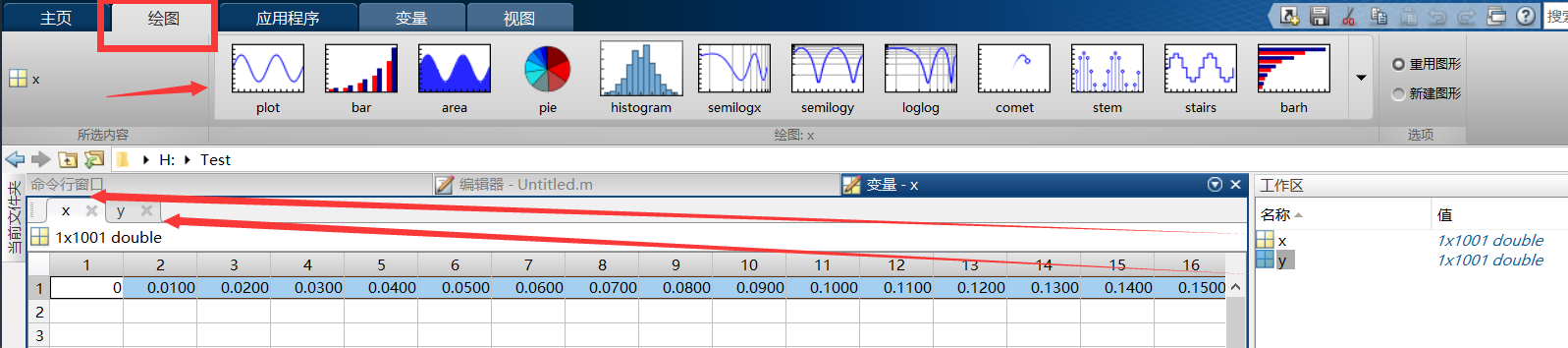我们这里，选中第1节输入的数据变量y，然后点击“plot”工具箱，绘图结果如下图所示，出现了一条美丽的正弦曲线。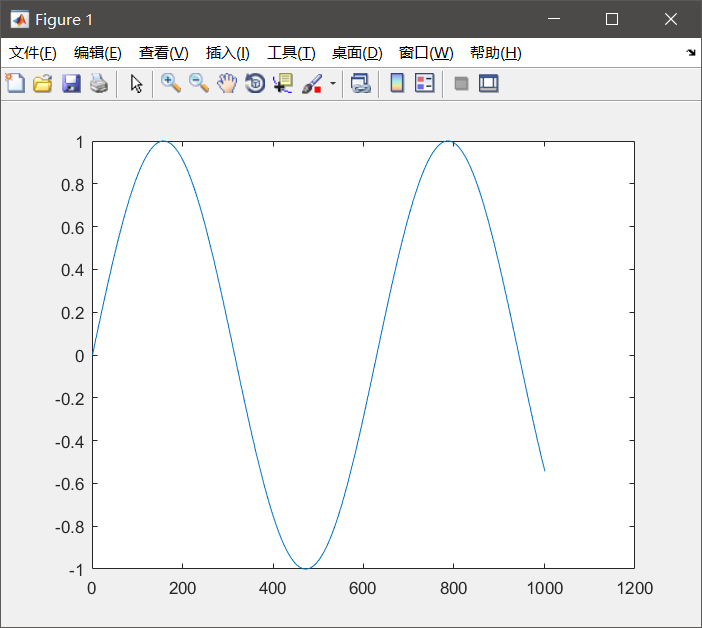在图片上添加信息，点击“插入”，选择要添加的内容选项。这里选择的x轴的标签，内容为“T”。
除此之外，还可以添加“Y标签”、“标题”、“图例”、“颜色栏”、“线”、“箭头”、“文本箭头”、“双箭头”、“文本框”、”矩形“、”椭圆“、”坐标轴“、”灯光“等内容。
如何使用，大家可以自行探索。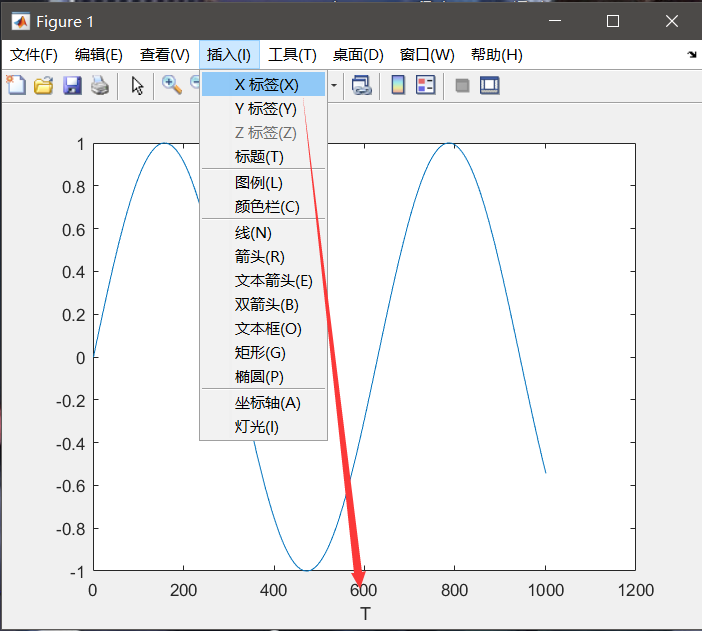按照以上方法，依次试用其他绘图工具箱
2.2线图
semilogx工具箱（x轴半对数)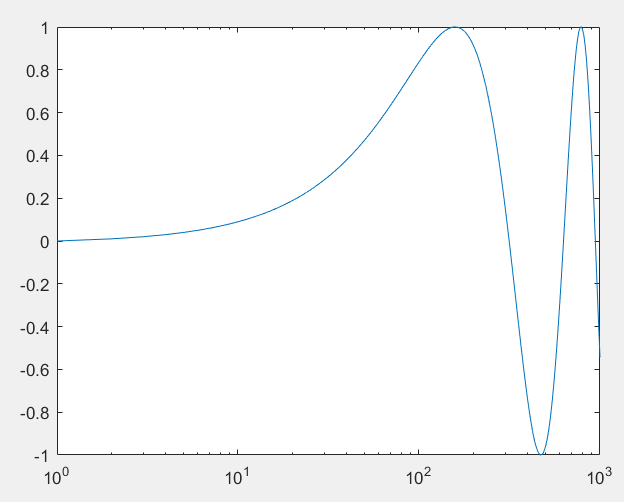semilogy工具箱（y轴半对数)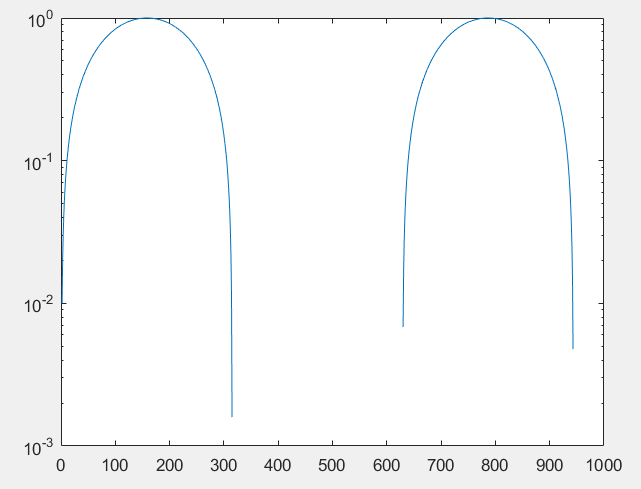loglog工具箱（xy轴都取对数）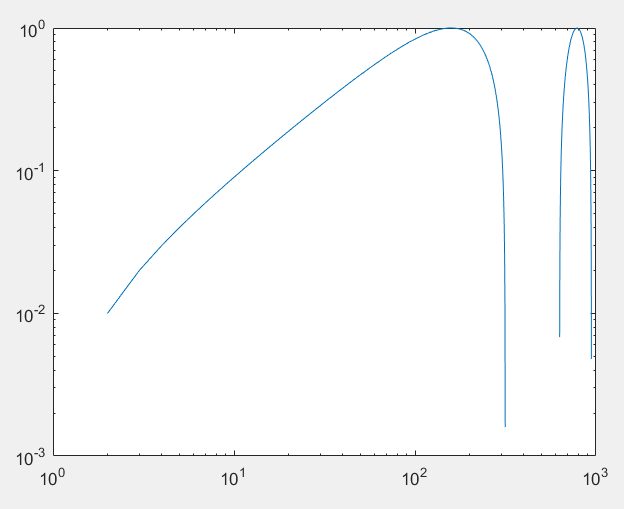area工具箱（区域填充图）comet工具箱（可以清楚地显示画图轨迹，如彗星飞行一般）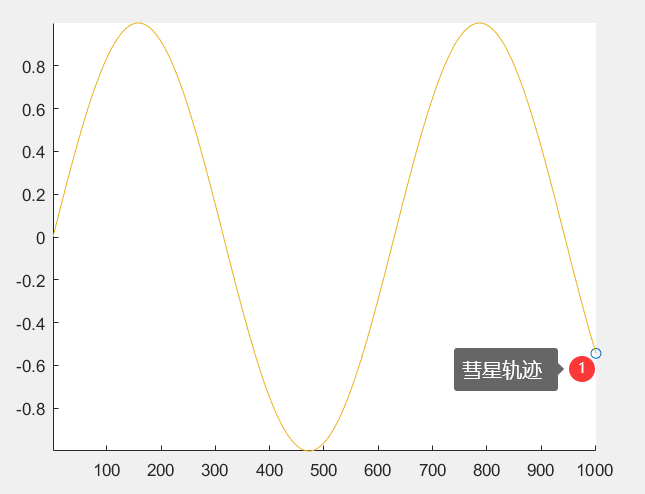2.3 针状图与阶梯图
stem工具箱（离散序列或者针状图）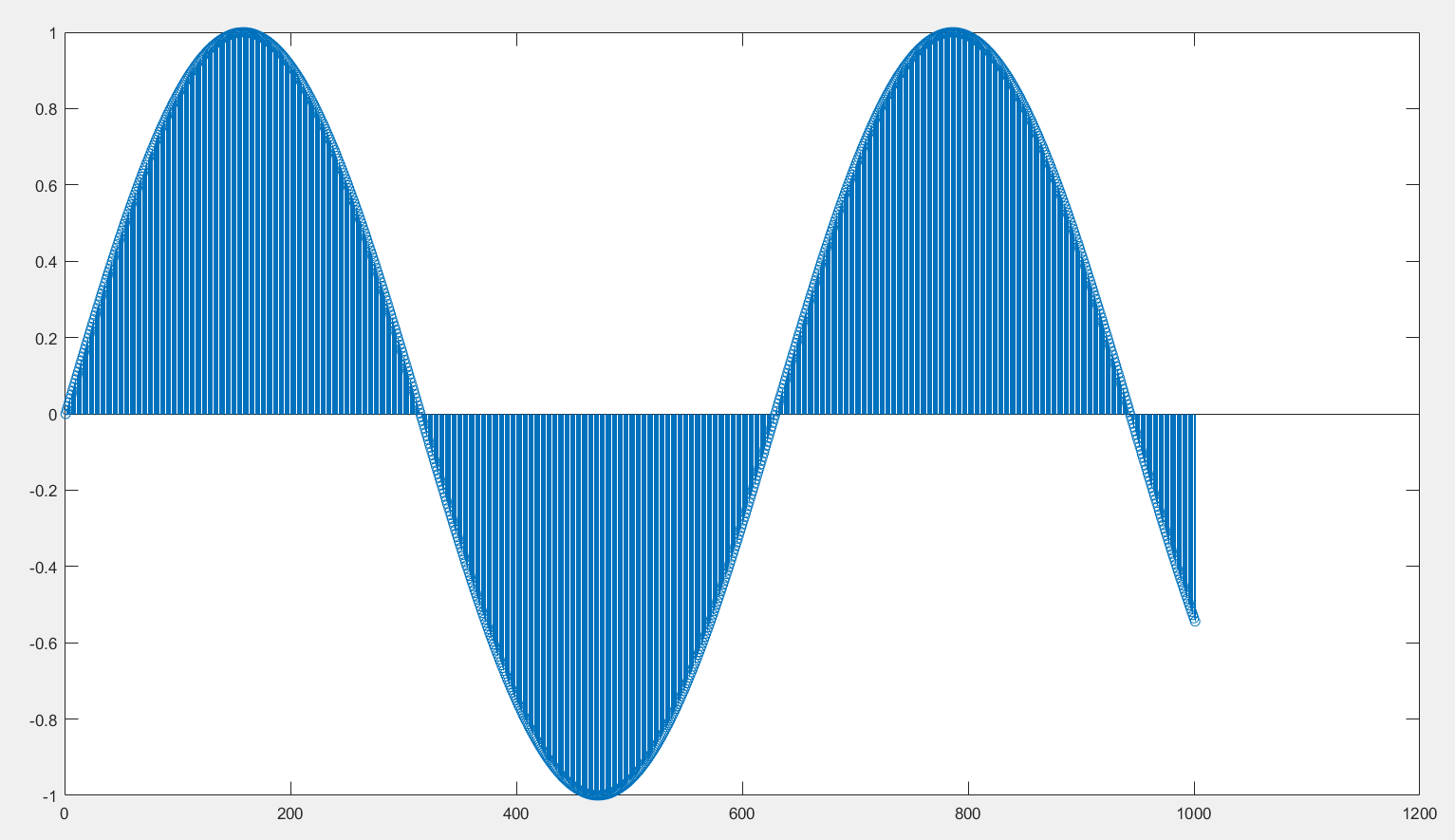stairs工具箱（阶梯图）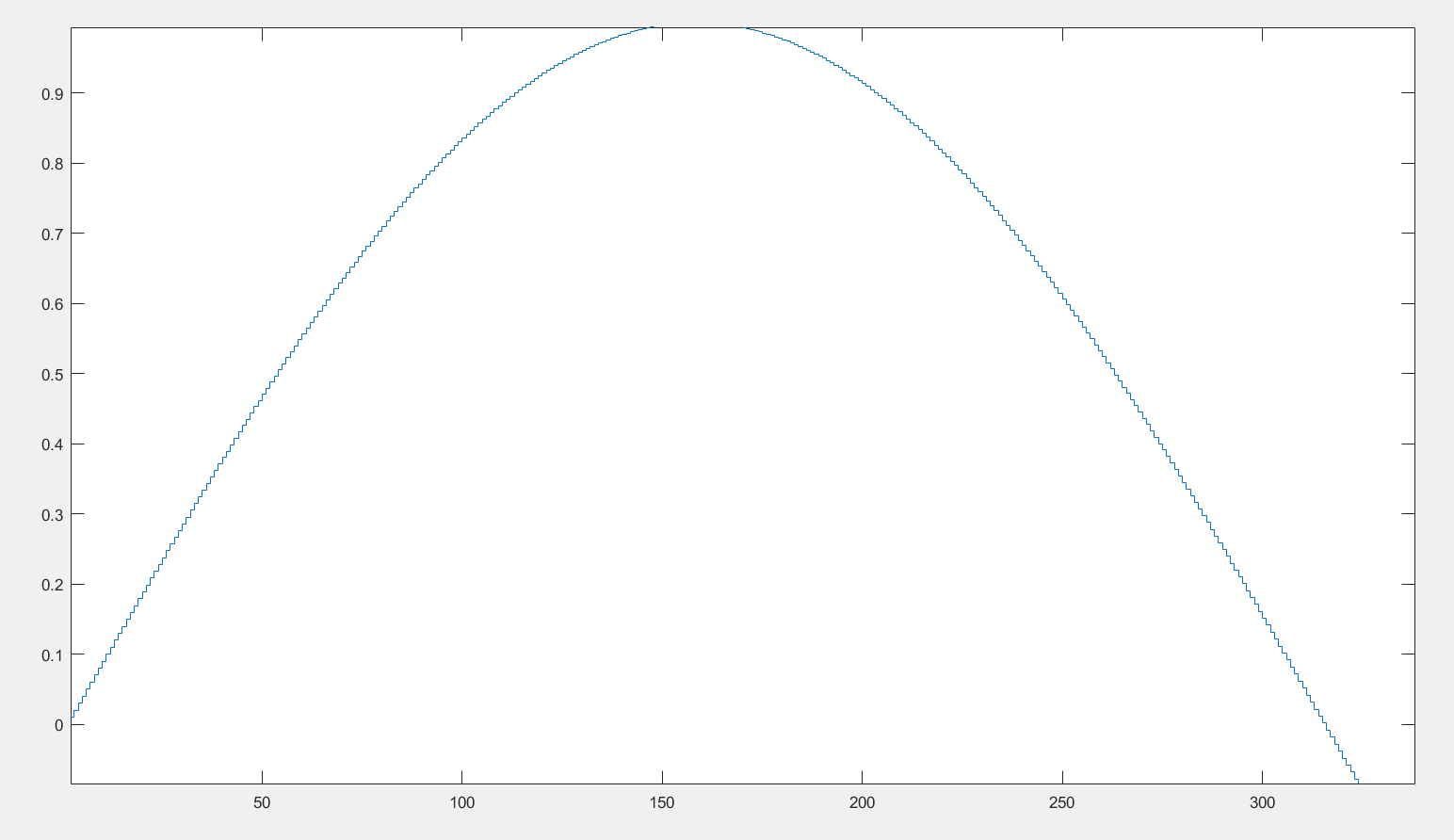2.4条形图
bar工具箱（条状图）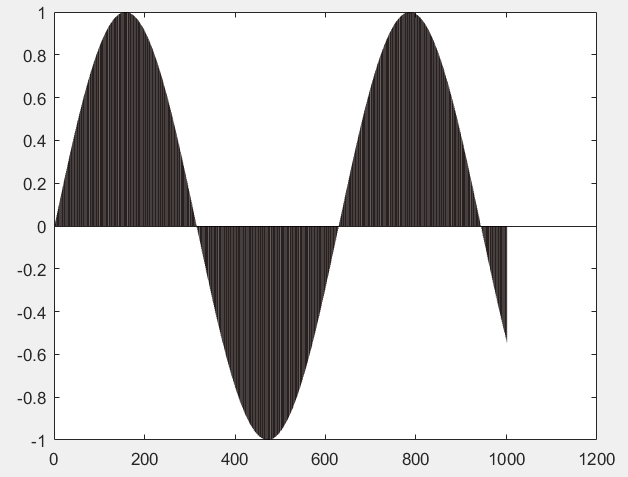barh工具箱（水平条状图）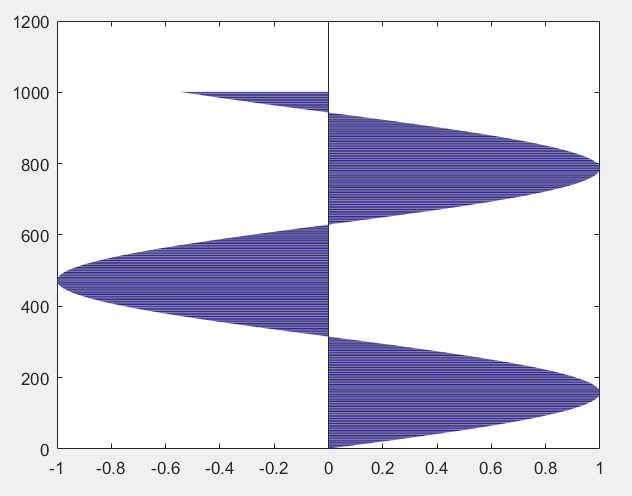histogram工具箱（直方图）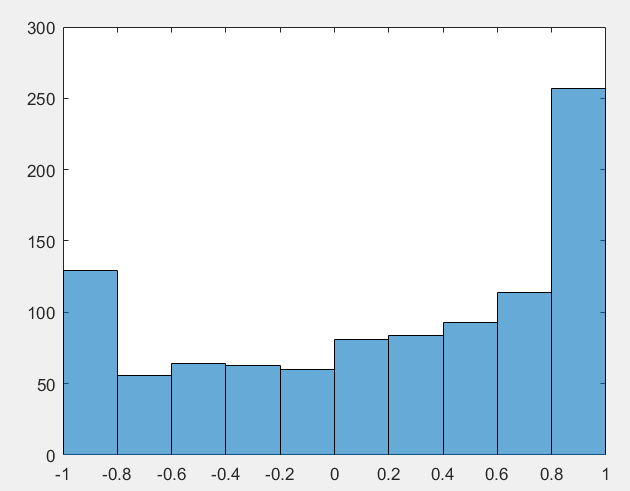pareto工具箱（泊拉图）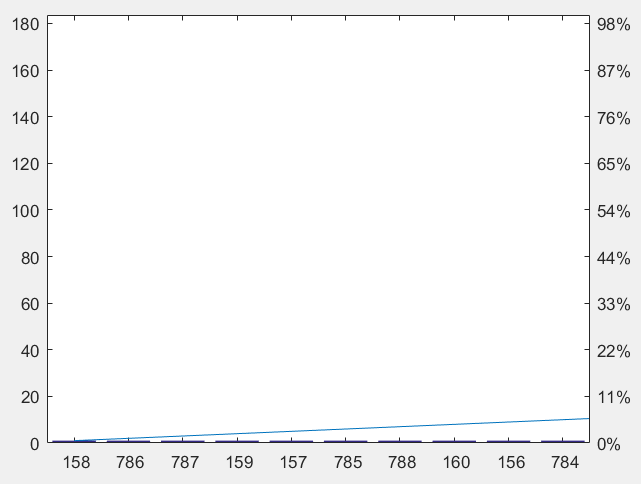bar3工具箱（三维条形图）
load count.dat
Y = count(1:10,:);

%%
% Create a 3-D bar graph of Y and set the bar width to 0.5.
width = 0.5;

figure
bar3(Y,width)
title('Bar Width of 0.5')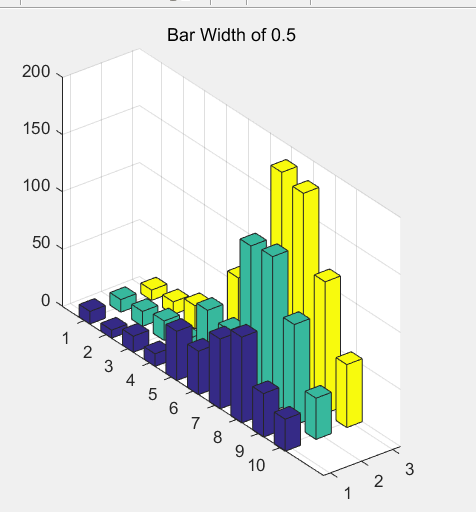bar3h工具箱（三维水平条形图）
load count.dat
Y = count(1:10,:);

%%
% Create a 3-D horizontal bar graph of |Y|. By default, the style is
% |detached|.
figure
bar3h(Y)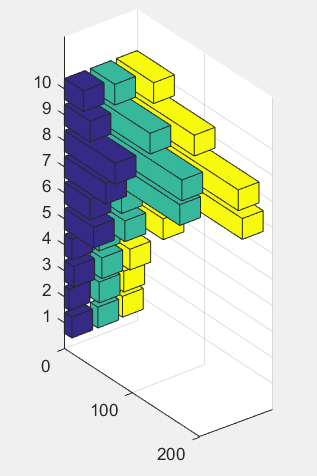2.5散点图
spy工具箱（可视化稀疏模式）
B = bucky;
spy(B)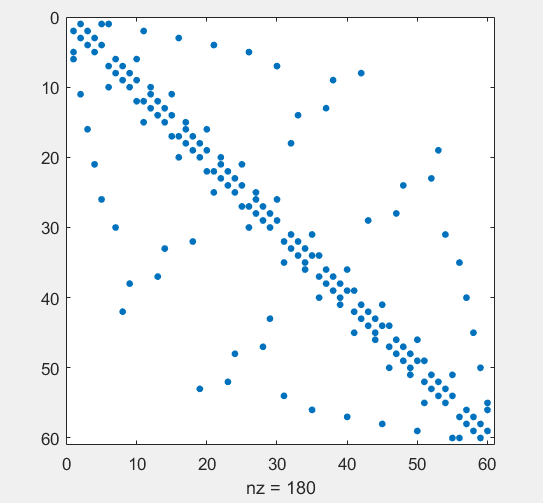2.6饼图
pie工具箱（饼图）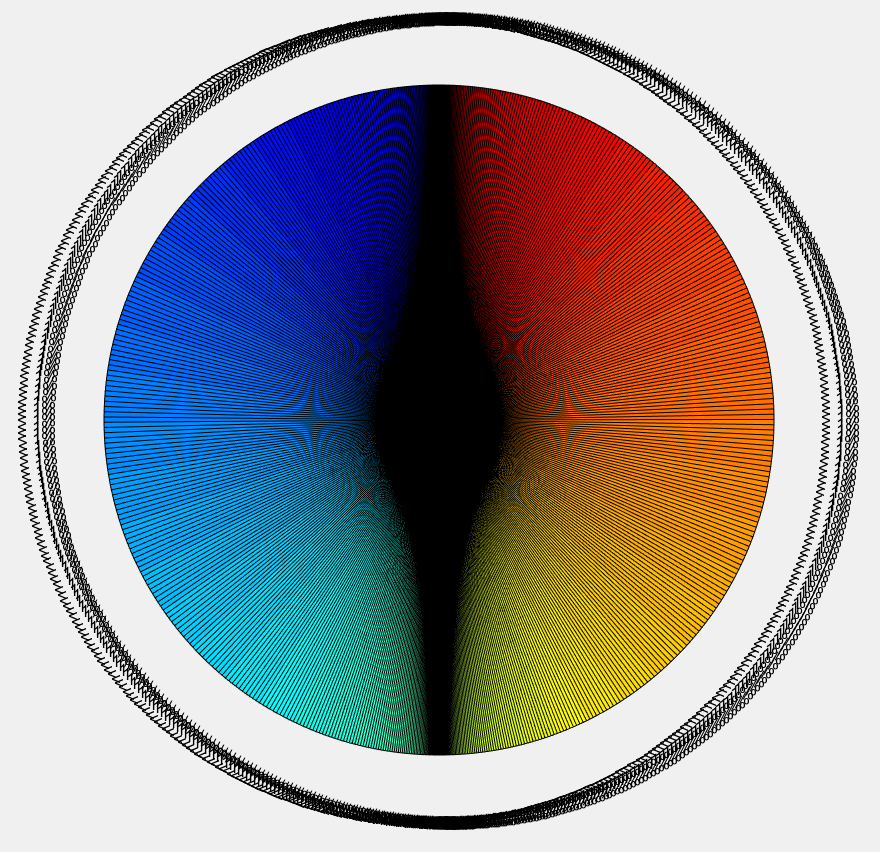pie3工具箱（三维饼图）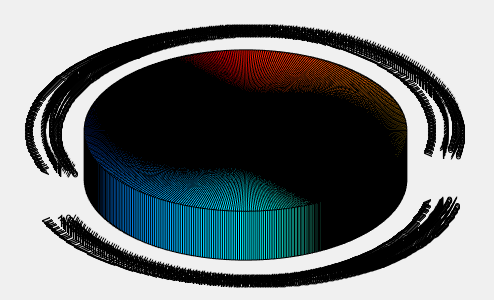2.7直方图
histogram工具箱（直方图）rose工具箱（角直方图）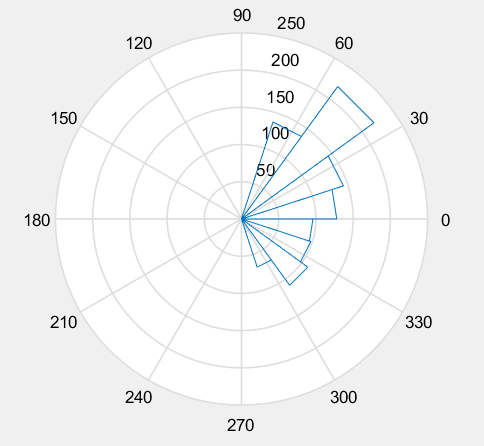2.8极坐标图
polar工具箱（极坐标图）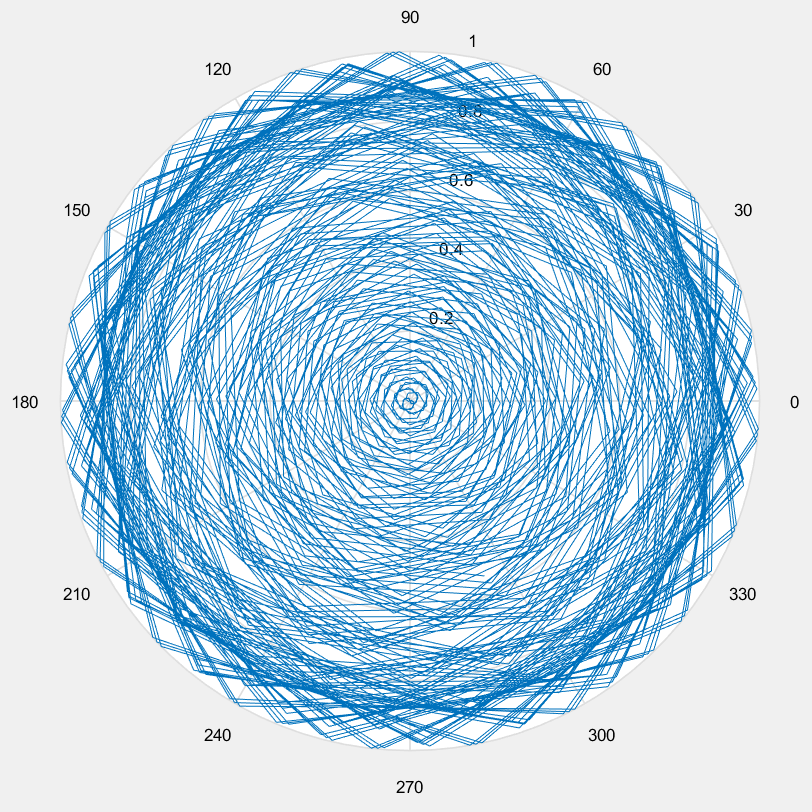rose工具箱（角直方图）compass工具箱（罗盘图）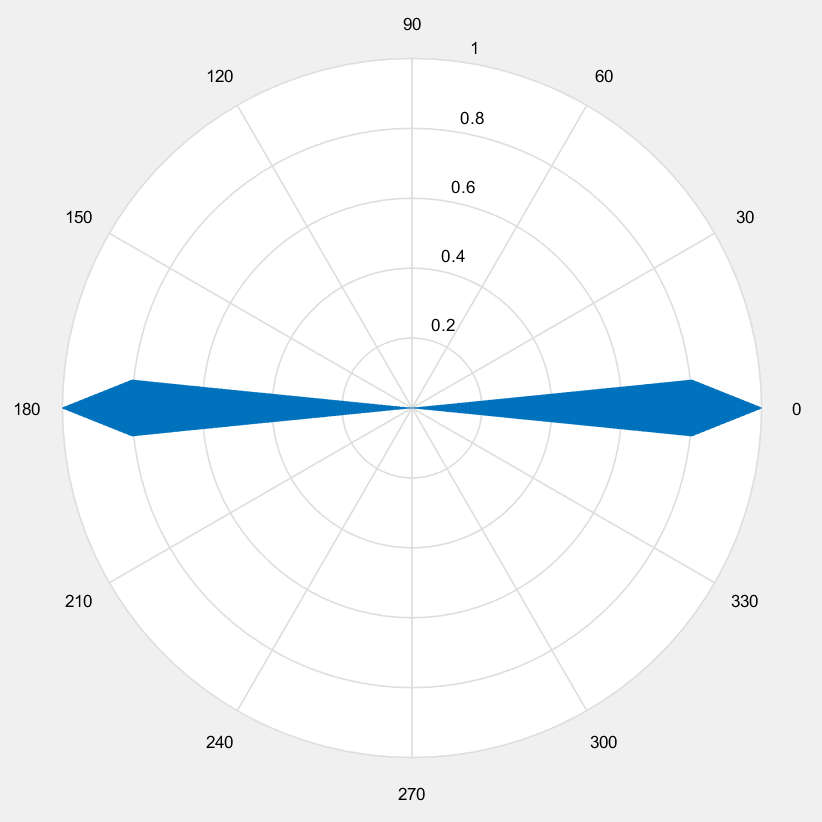2.9矢量场图
feather工具箱（羽状图）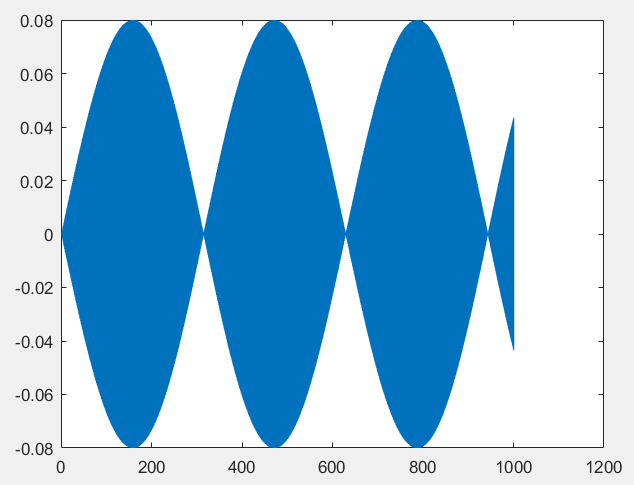compass工具箱（罗盘图）2.10窗口可视化工具（信号处理工具箱）
wvtool工具箱（窗口可视化工具）信号处理中，时域、频域图，应该会用到。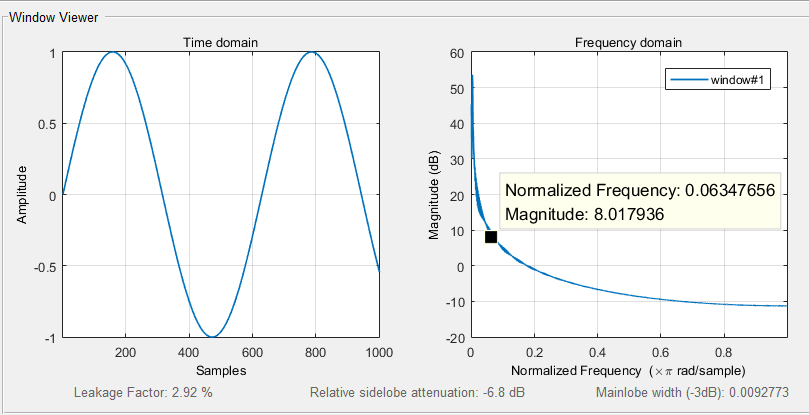periodogram-psd工具箱(使用周期法进行PSD估计)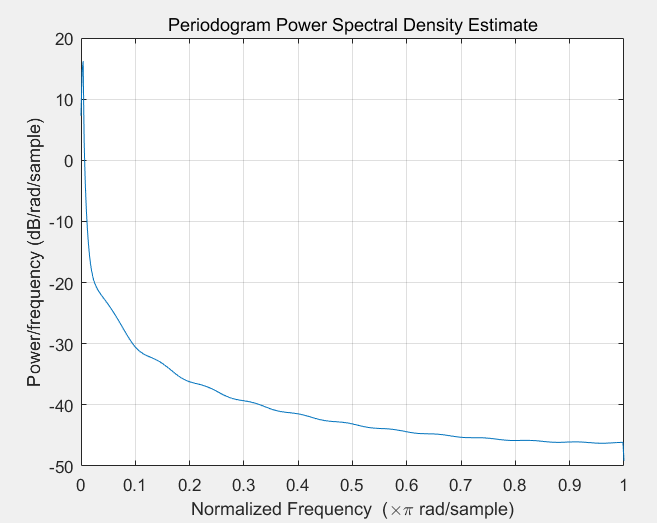periodogram-power工具箱（使用周期法进行功率谱估计）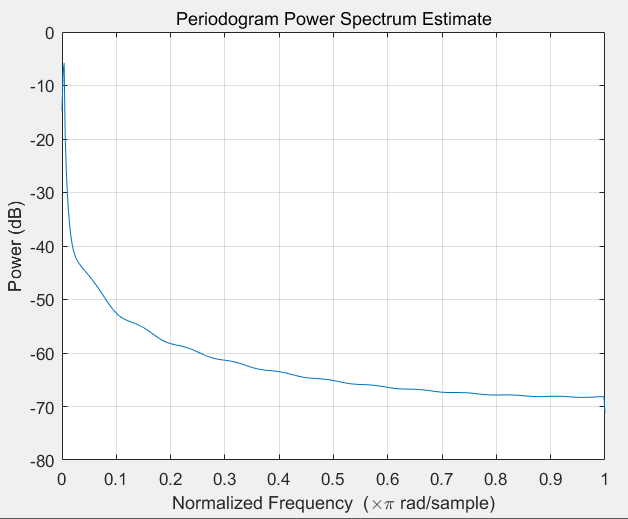pwelch-psd工具箱（使用welch进行PSD估计）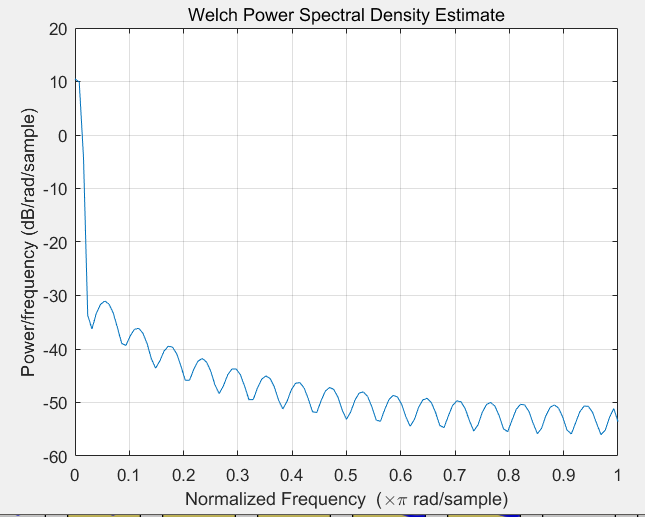pwelch-power工具箱（使用welch进行功率谱估计）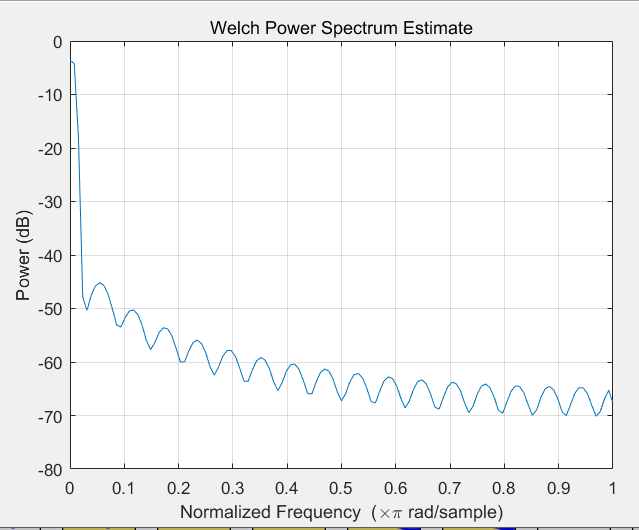pmtm工具箱（使用多窗谱（MTM）计算psd）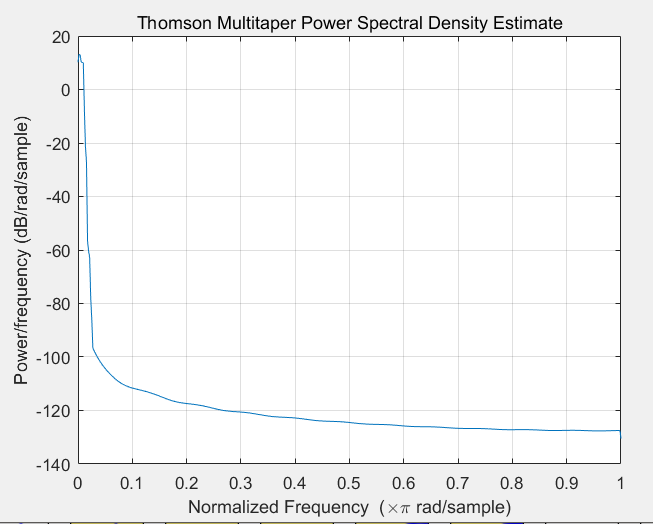pburg工具箱（使用burg法计算psd）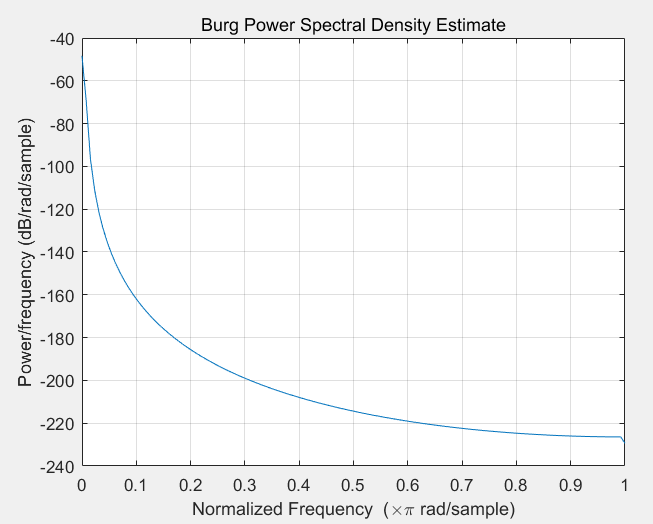pcov工具箱（使用协方差法cov计算psd）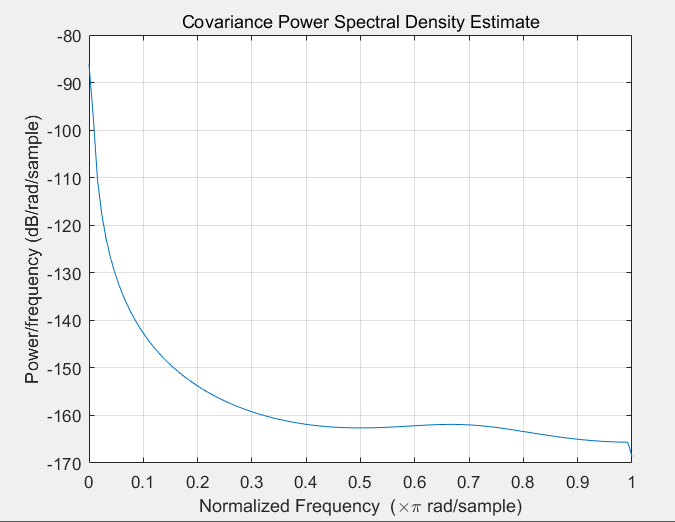pmcov工具箱（使用修正协方差法mcov计算psd）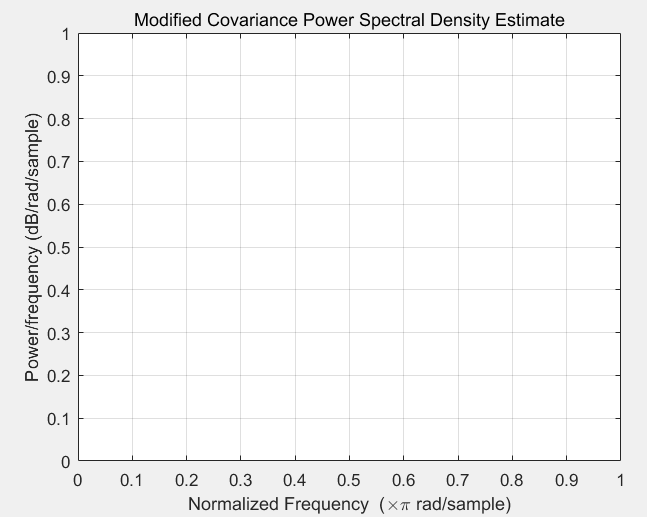pyulear工具箱（使用yule walker AR法计算psd）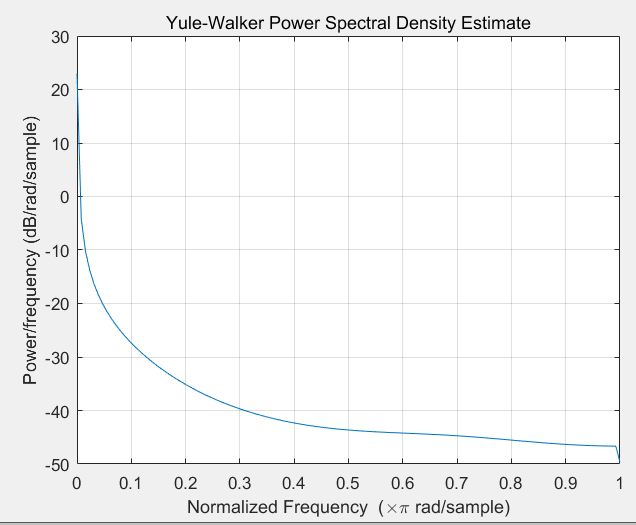peig工具箱（使用 特征矢量法的伪频谱）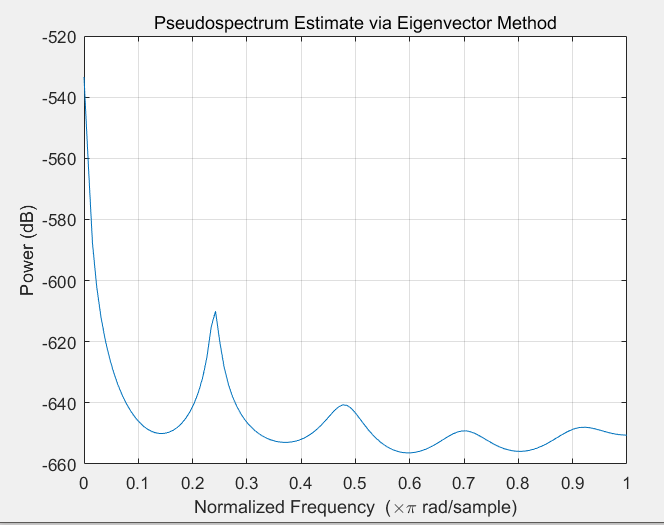pmusic工具箱（使用music计算的伪频谱）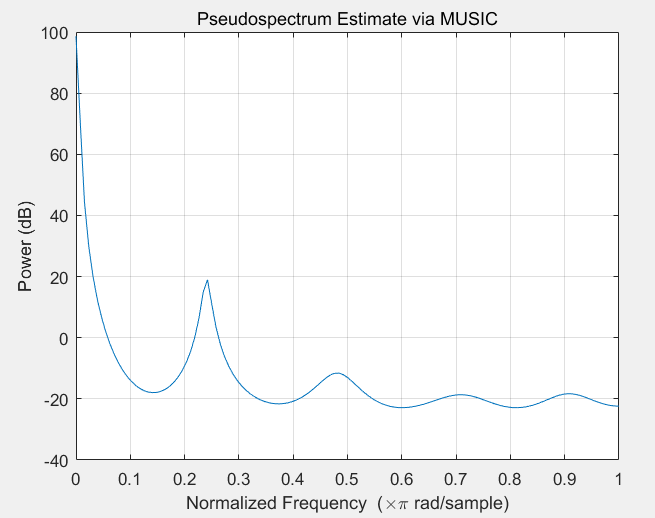spectrogram工具箱（使用短时傅里叶变化的频谱图）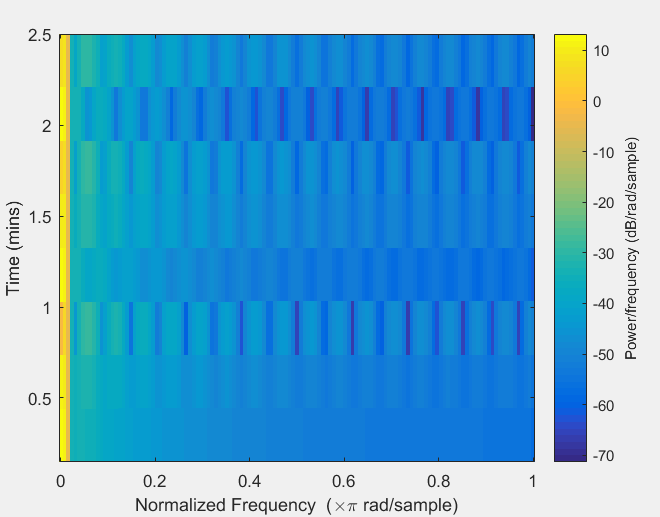strips工具箱（带状图）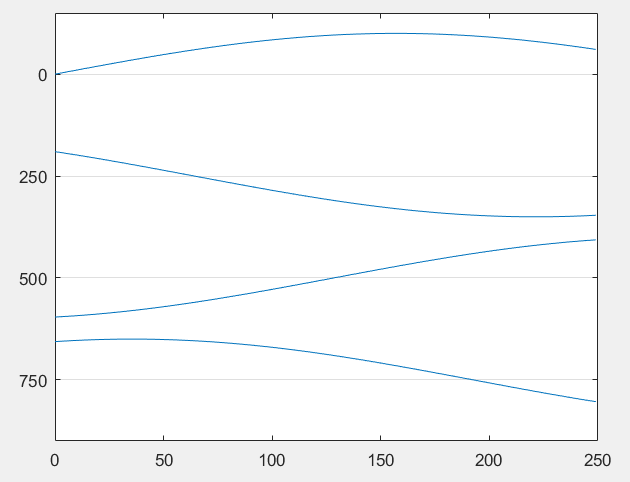2.11统计和机器学习工具箱
boxplot工具箱（箱线图）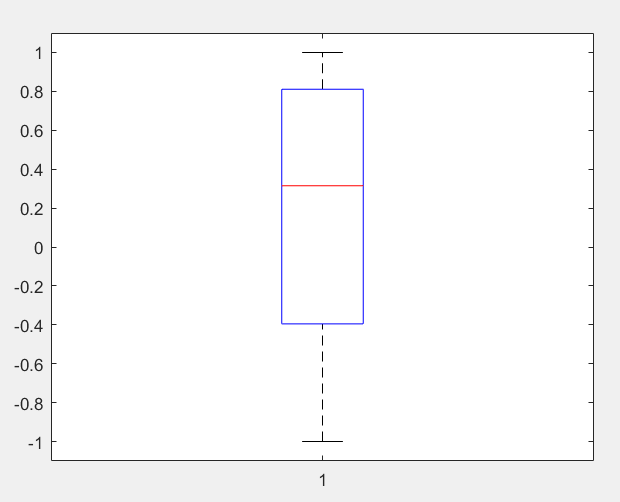ecdf工具箱（经验累积分布函数）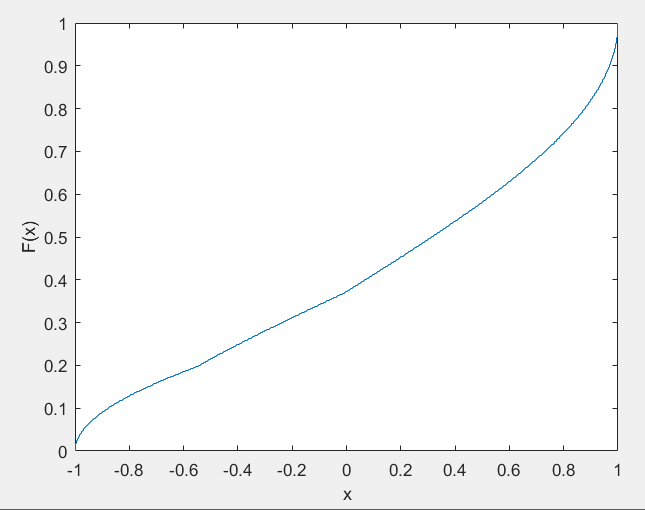histfit工具箱（正常拟合的直方图）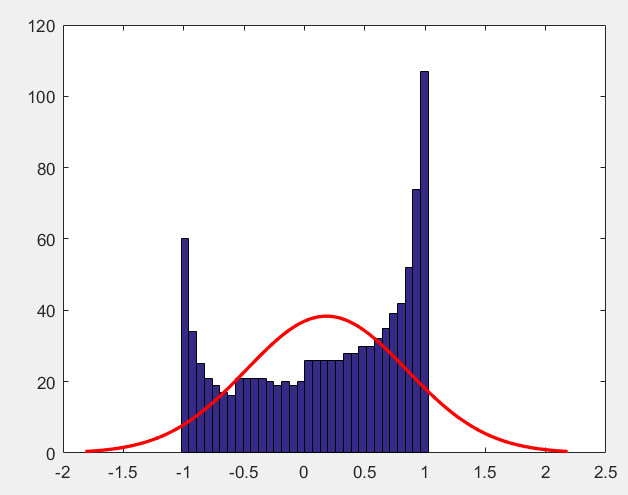ksdensity工具箱（核平滑密度估计）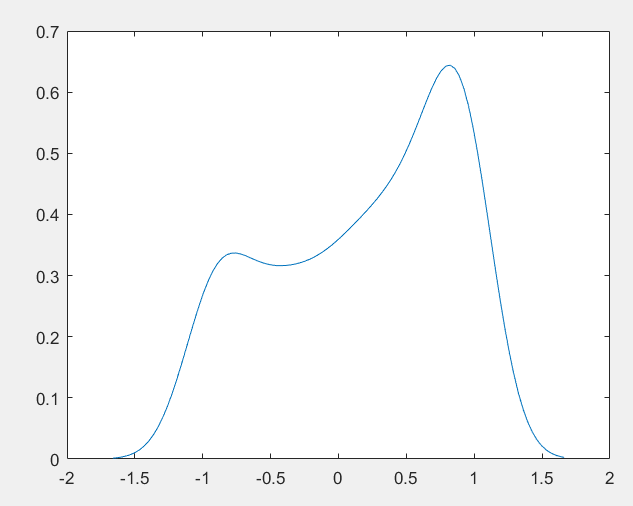probplot工具箱（正态概率图）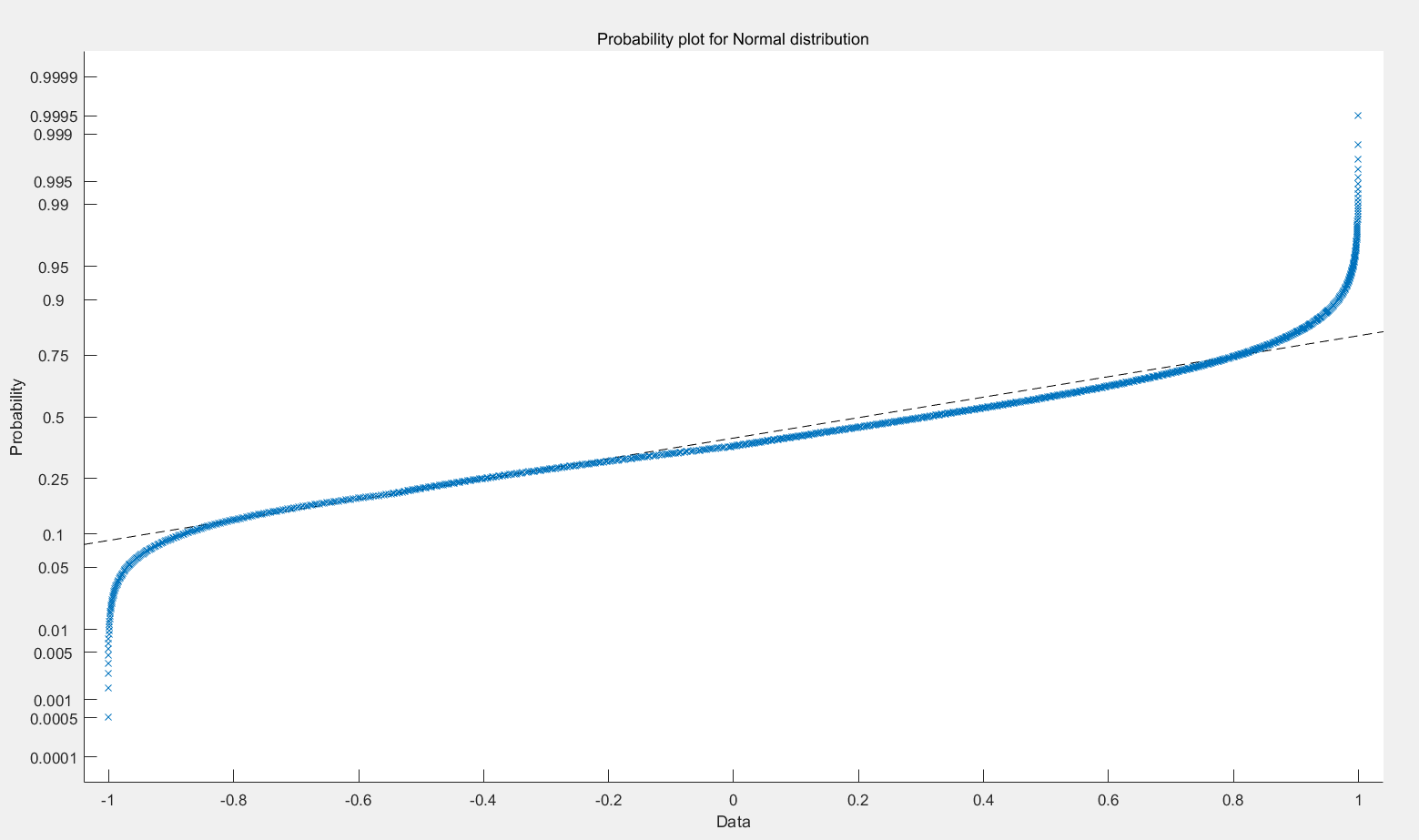qqplot工具箱（分位数图）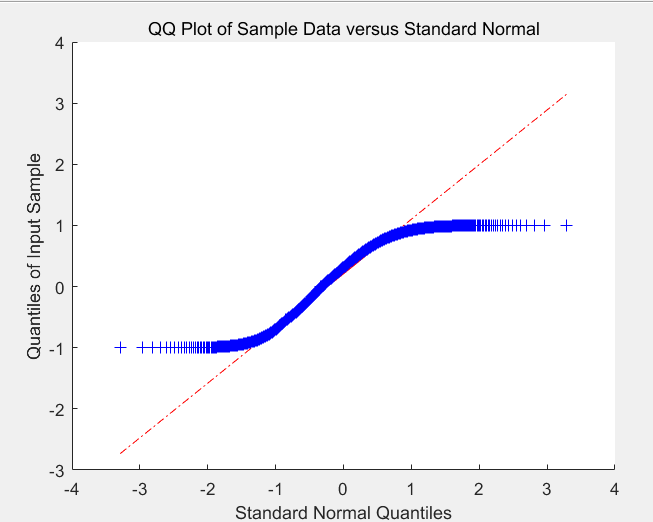andrewsplot工具箱（安德鲁斯）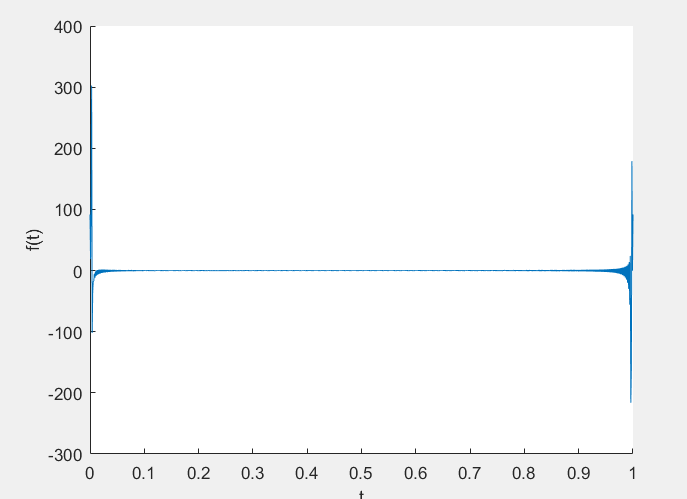glyphplot工具箱（字形图）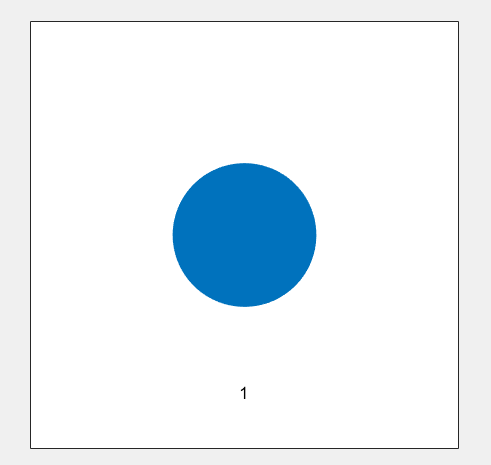展开全文可视化 数据可视化
• matlab开发-多相立体图像分割。根据不同的公式进行多相水平集图像分割
•opencv 计算机视觉 线性代数 矩阵
• 下面来介绍一下如何在MATLAB完成三维立体图像的制作，具体如下： 1、在MATLAB的主界面编辑器中写入下列代码： 三维曲线程序： clear all;%%表示清空所有变量及变量值%% t=0:pi/360:2*pi; x=sin(t); y=cos(t); ...
• 通过matlab标定和立体校正，加载左右图片，保存标定参数，然后对左右图片进行立体校正，具体效果我博客有介绍。
• matlab开发-高光谱图像立体切片机。图像切片器，用于可视化多通道图像数据。
• 动态关键的两个函数 set（）和drawnow 第一步，先表示出静态 第二步，循环来画出更新后的图像，用set（）来更新数据，用drawnow来更新图像 例子一， %第一步% x= 0:pi/50:2*pi; y=sin(x); h=plot(x,y) %第...
• 我原以为理解并且会应用matlab绘制三维，但动起手来，我发现各种不会，弄着弄着都有点怀疑人生了。首先，plot与plot3分别绘制二维与三维的曲线，而不是曲面，这是我的一个误区！还有，当你有x，y，z数据（行向量...
• 立体图：随机点立体图 stereogram(A)，其中 A 是单色图像，绘制 A 的随机点立体图 (RDS)。 A 应定义为双精度矩阵，但具有整数值。 在立体图中，具有正值的 A 区域看起来几乎在屏幕前面，而具有负值的区域似乎在屏幕...
• STEREOVIEW 立体绘图功能 立体视图抓取当前的 3D 图形并将其绘制成两个立体图像。 启动后，您可以： 1）选择眼间距系数（idc） 定义为与相机的距离之间的比率目标与两眼距离； 值 30 工作正常。 2) 选择查看模式：...
• 在科学目视表示（Scientific visualization）中，三度空间的立体图是一个非常重要的技巧。本章将介绍MATLAB基本XYZ三度空间的各项绘图命令。 mesh和plot是三度空间立体绘图的基本命令，mesh可画出立体网状图，plot则...
• ## MATLAB画心形立体图

万次阅读 多人点赞 2018-04-23 08:47:45
代码如下&gt;&gt; [x,y,z]=meshgrid(linspace(-3,3,120)); f=(x.^2+(9*y.^2)./4+z.^2-1).^3-((9*y.^2).*(z.^3))./80-(x.^2).*(z.^3); p=patch(isosurface(x,y,z,f,0)); set(p,'FaceColor','r') grid on...
• 主要介绍了matlab画三维图像的示例代码(附demo),文中通过示例代码介绍的非常详细，对大家的学习或者工作具有一定的参考学习价值，需要的朋友们下面随着小编来一起学习学习吧
• 该函数为未校准（几乎对齐）的立体对生成整流单应性。
• 立体图 - 显示由两个立体图像获得的立体图 不带参数的浮雕，打开一个对话框以选择包含单个图像文件中的两个立体图像。 anaglyph('filename') 显示图像“文件名”的立体图（带扩展名），其中包含两个立体图像图像...
• 在此文件中，使用了 mri.mat 数据。 如果要显示其他数据，可以将数据保存到矩阵 D1，或修改 .m 文件。 单击任何三个 MRI 图像，然后按“ENTER”以在新的 [x,y,z] 位置更改切片。 按“ESC”并按“ENTER”退出。
• 红色为月均值。蓝线代表每年的月序列。 主要命令通过plot3实现，在画侧面的时候，仍采用plot3命令，但是年份轴要取一个恒定值（2019）。代码有时间再整理。 【1】添加置信区间
• 这个MatLab工具箱允许绘制带有浮雕注释的函数。 用户还可以控制注释的深度。 添加一名作者电子邮箱：Illia.ihnatenko@student.tuke.sk 斯洛伐克科希策工业大学学生。
• 第一章 二维图形的绘制 § 基本绘图函数 § 绘图入门 § 图形窗口的分割 § 图形窗口的修饰 § 绘制矩阵 第二章 特殊二维绘图函数 第三章 三维图形绘制 § 绘制三维曲线 ...§ 绘制三维曲面...

# matlab立体图matlab 订阅